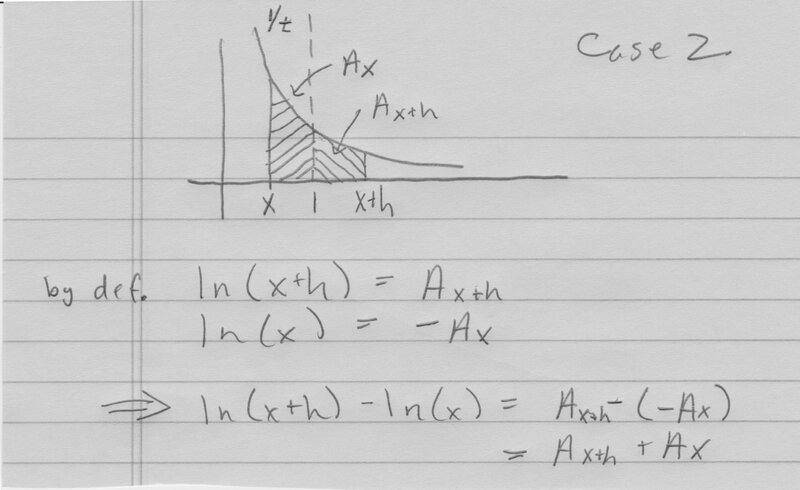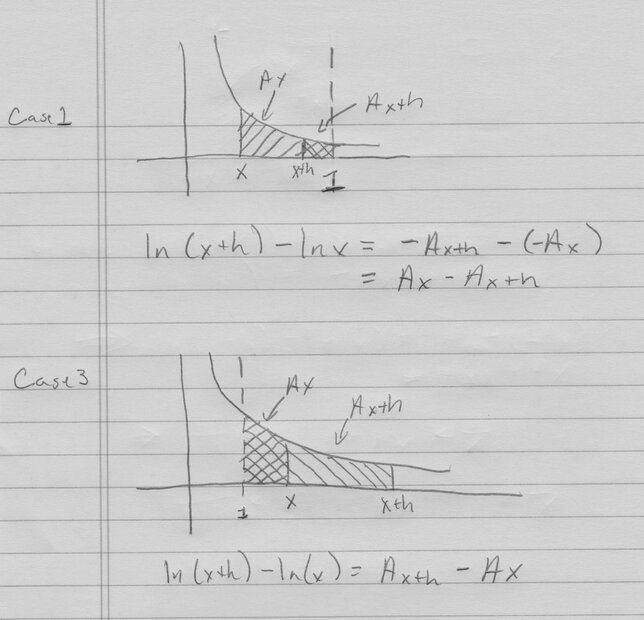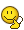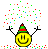# How to conclude a mathematical expression with the provided background

Gold Member
Hi PF

In my textbook, the Spanish 6th edition of "Calculus", by Robert A. Adams, at Chapter 3.2, Theorem 1 states:

If ##x>0##.

$$\dfrac{d}{dx}\ln{x}=\dfrac{1}{x}$$

PROOF For ##x>0## and ##h>0##, ##\ln{(x+h)}-\ln{(x)}## is the area of the shaded part (...) Regard the shaded area at Figure 3.10All of this is right. My question is: let's put I know nothing but what I've read until this page: could anybody conclude that ##\ln{(x+h)}-\ln{(x)}## is the area pointed out? As far as I know, the starting point is the Definition 6 of natural logarithm:

"For ##x>0##, be ##A_x## the flat area bounded by ##y=1/t##, the ##t## axis, ##t=1## and ##t=x##. Function ##\ln x## is defined this way:

$$\ln x=\begin{cases}{A_x}&\text{if}& x\geq{1}\\-A_x & \text{if}& 0<x<1\end{cases}$$

As shown in Figure 3.9Greetings

Last edited by a moderator:

jedishrfu
Mentor
I'm not sure where you are heading with your question. Could you elaborate a bit more? Is it that you question the area being ln(x)?

Last edited:
•mcastillo356
Gold Member
Yes, ##0<x<1## for the ##-A_x## (mentor note: edited first post to fix this typo)

I distinguish three possible cases, based on the definition:

1- For ##1>x>0## and ##1>h>0##, the area bounded by ##y=1/t##, ##y=0##, and ##t=x## y ##t=x+h##, would be ##-(\mbox{ln}\;(x+h)+\mbox{ln}\;x)##;

2- for ##x>1## and ##1>h>0##, ##\mbox{ln}\;(x+h)-\mbox{ln}\;x##;

3- for ##x>1## and ##h>1##, ##\mbox{ln}\;(x+h)+\mbox{ln}\;x##.

Last edited by a moderator:
Mark44
Mentor
My question is: let's put I know nothing but what I've read until this page: could anybody conclude that ##\ln{(x+h)}-\ln{(x)}## is the area pointed out?
I'm not sure. In your textbook, has it already been proven that ##\int_a^b \frac {dt} t = \ln (b) - \ln(a)##? It seems to me that the author is using this fact to conclude that the area shown is ##\ln(x + h) - \ln(x)##.

•jedishrfu
Gold Member
No trace of Riemann, or Fundamental Theorem of Calculus, needed to face this conclusion. But... I've got algebra:
Instead of ##f(x)=1/t##, let's imagine ##f(x)=1##. Be ##g:=f(x)(x-1)##. We have ##g(1)=0##.
If ##x>1##, ##g(x)## is the area below ##f(x)## from 1 to ##x##. If ##0<x<1##, ##g(x)=f(x)(x-1)=-f(x)(1-x)##, minus the area below ##f(x)## from ##x## to 1? What does it mean this last sentence? How do I use this example to explain ##\ln(x+h)-\ln (x)##?PeroK
Homework Helper
Gold Member
2020 Award
I would imagine the first step is to prove that ##\frac{d}{dx}\ln x = \frac 1 x## using the chain rule; then, using the FTC show that the antiderivative of ##\frac 1 x## is ##\ln | x |+ C##.

I don't understand at all what the book is doing.

•mcastillo356
Gaussian97
Homework Helper
I think you are overcomplicating everything, just from the definition of ln (as given in the book) the property follows trivially, @mcastillo356 is not far when doing
I distinguish three possible cases, based on the definition:

1- For ##1>x>0## and ##1>h>0##, the area bounded by ##y=1/t##, ##y=0##, and ##t=x## y ##t=x+h##, would be ##-(\mbox{ln}\;(x+h)+\mbox{ln}\;x)##;

2- for ##x>1## and ##1>h>0##, ##\mbox{ln}\;(x+h)-\mbox{ln}\;x##;

3- for ##x>1## and ##h>1##, ##\mbox{ln}\;(x+h)+\mbox{ln}\;x##.
But, first of all, your three cases are not really good, for example the two possibilities x=0.5, h={0.1, 0.6} are both in case 1, but ##\ln{(x+h)}## is defined differently for each case because ##x+h=0.6<1## in the first case but ##x+h=1.1\geq1## in the second one.
So let's see if you can split the ##x## and ##h## space in a better way.
Then you should also look more carefully at the definition of ln (splitting into cases correctly help you with this) and you will see that indeed the area they tell you is exactly equal to the difference ##\ln{x+h}-\ln{x}##.

••jedishrfu and mcastillo356
Gold Member
Hello
This post is written as a doubt, a question, rather than a statement . I start quoting the book, and then comes my attempt to fit the mathematical expression ##\ln (x+h)-\ln x## with the context.
"DEFINITION 6 Natural logarithm
For ##x>0##, be ##A_x## the flat area bounded by the curve ##y=1/t##, the ##t## axis and ##t=1##, and ##t=x##. The function ##\ln x## is defined this way:
$$\ln x\begin{cases}{A_x}&\text{if}& x\geq{1}\\-A_x & \text{if}& 0<x<1\end{cases}$$
shown in this figure(a)##\ln x=-\mbox{area}\;A_x##, if ##0<x<1##
(b)##\ln x=\mbox{area}\;A_x##, if ##x\geq{1}##
The definition implies ##\ln 1=0##; ##\ln x>0## if ##x>1##; ##\ln x<0## if ##0<x<1## and is a one-to-one function. Now we will prove that if ##y=\ln x##, then ##y'=1/x##. The proof of this result is similar to the proof of the Theorem Fundamental of Calculus (...)
THEOREM 1 If ##x>0## then
$$\frac{d}{dx}\ln x=\dfrac{1}{x}$$
PROOF For ##x>0## and ##h>0##, ##\ln(x+h)-\ln x## is the flat area bounded by ##y=1/t##, ##y=0##, and vertical lines ##t=x## and ##t=x+h##. Regard the shaded area shown in this figure..."My try:
For ##1>x>0## and ##1>h>0##
##-\ln x<0##
##-\ln (x+h)<0##
$$-(\ln (x+h)+\ln x)<0$$
For ##x>1## and ##1>h>0##
##\ln x>0##
##1<\ln (x+h)<0##
$$\ln (x+h)<\ln x$$
For ##x>1## and ##h>1##
##\ln x>0##
##\ln (x+h)>0##
$$\ln (x+h)>\ln x$$

Greetings

Gaussian97
Homework Helper
My try:
For ##1>x>0## and ##1>h>0##
##-\ln x<0##
##-\ln (x+h)<0##
$$-(\ln (x+h)+\ln x)<0$$
This is not true, you yourself have noticed few lines before that ##0<x<1 \Longrightarrow \ln{x}<0## but now you're saying that ##0<x<1 \Longrightarrow -\ln{x}<0##, how could that be possible?
I have already given you an argument why ##-\ln (x+h)<0## is false in my previous post.
Therefore clearly your final expression is wrong.

For ##x>1## and ##1>h>0##
##\ln x>0##
##1<\ln (x+h)<0##
$$\ln (x+h)<\ln x$$
Does ##1<\ln (x+h)<0## even make sense?
For ##x>1## and ##h>1##
##\ln x>0##
##\ln (x+h)>0##
$$\ln (x+h)>\ln x$$
The first two are correct, but writing one statement below another without any connection is not a valid proof in mathematics.

I would recommend you to read again my previous post.

•mcastillo356
Delta2
Homework Helper
Gold Member
I'm not sure. In your textbook, has it already been proven that ##\int_a^b \frac {dt} t = \ln (b) - \ln(a)##? It seems to me that the author is using this fact to conclude that the area shown is ##\ln(x + h) - \ln(x)##.
The way I understand it is that the book, essentially uses the equation $$\int_1^x \frac{dt}{t}=\ln x-\ln1$$ as the definition of the function ##f(x)=\ln x##.

•mcastillo356
Mark44
Mentor
The way I understand it is that the book, essentially uses the equation $$\int_1^x \frac{dt}{t}=\ln x-\ln1$$ as the definition of the function ##f(x)=\ln x##.
That's more or less what I was asking about in post #4...

•mcastillo356 and Delta2
caz
Gold Member
There are 3 cases (x,h are both greater than zero)
x<1,x+h<1
x<1,x+h≥1
x≥1,x+h≥1
Try drawing a picture for each of the cases of the areas under the 1/x curve from the definition of ln

•Delta2
Gold Member
There are 3 cases (x,h are both greater than zero)
x<1,x+h<1
x<1,x+h≥1
x≥1,x+h≥1
Try drawing a picture for each of the cases of the areas under the 1/x curve from the definition of ln
Hello, PF
I've drawn each case with Geogebra, but I've had to use, for example and for the first case, ##x<1## and ##(x+h)<1## the command "Integral(1/x,0.6,0.8)", and so on.
Let's write down the text again (the following is to read over...)
DEFINITION 6 The natural logarithm
For ##x>0##, be ##A_x## the shaded flat surface bounded by ##y=1/t## curve, the ##t## axis and the vertical line ##t=1## and ##t=x##. ##\ln x## function is defined this way:

$$\ln x\begin{cases}{A_x}&\text{if}& x\geq{1}\\-A_x & \text{if}& 0<x<1\end{cases}$$

shown in Figure 3.9

The definition implies that for ##\ln 1=0##, ##\ln x>0## if ##x>1##, ##\ln x<0## if ##0<x<1##, and is a one-to-one function. We will prove that if ##y=\ln x##, then ##y'=1/x##. The proof of this theorem is similar to the demonstration of the TFC we will provide in section 5.5.

THEOREM 1 If ##x>0##, then

$$\dfrac{d}{dx}\ln x=\dfrac{1}{x}$$

DEMONSTRATION For ##x>0## and ##h>0##, ##\ln(x+h)-\ln x## is the area of the flat surface bounded by ##y=1/t##, ##y=0##, and the vertical lines ##t=x## and ##t=x+h##. Belongs to the shaded area in Figure 3.10. Comparing this area with the one of the two rectangles it follows that

$$\dfrac{h}{h+x}<\mbox{shaded area}=\ln(x+h)-\ln x<\dfrac{h}{x}$$

(Then comes the well-known figure)...

The fact is that I'm not sure whether is it using Fundamental Theorem of Calculus or not, but I've done the homework (draw all the cases with Geogebra). Nothing works to conclude from the background that "For ##x>0## and ##h>0##, ##\ln(x+h)-\ln x## is the area of the flat surface bounded by ##y=1/t##, ##y=0##, and the vertical lines ##t=x## and ##t=x+h##", unless I say: ##\ln x## is a strictly increasing function. Then is trivial.

caz
Gold Member
I have a meeting in 5 minutes, so I’ll give a hint and check back later
ln(x+h) is the area under 1/x from 1 to x+h
ln(x) is the negative of the area under 1/x from x to 1
What is the difference between them?

edit: for case 2

Last edited:
•mcastillo356
caz
Gold Member
See pictureThe other two cases are similar

••Delta2 and mcastillo356
Gold Member
I disagree. It works your example...Let me see... Please, forgive me...I must think it over.
Thanks!caz
Gold Member
I disagree with your disagreement.Notice that there is an overlap region in both of these cases

Last edited:
•PhDeezNutz, mcastillo356 and Delta2
Gold MemberI have no words to express my gratitude.. Thanks!
Greetings

••PhDeezNutz, caz and Delta2